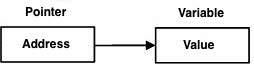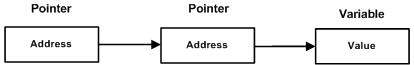# D Programming - Pointers

D programming pointers are easy and fun to learn. Some D programming tasks are performed more easily with pointers, and other D programming tasks, such as dynamic memory allocation, cannot be performed without them. A simple pointer is shown below.Instead of directly pointing to the variable, pointer points to the address of the variable. As you know every variable is a memory location and every memory location has its address defined which can be accessed using ampersand (&) operator which denotes an address in memory. Consider the following which prints the address of the variables defined −

```import std.stdio;

void main () {
int var1;

char var2;
}
```

When the above code is compiled and executed, it produces the following result −

```Address of var1 variable: 7FFF52691928
```

## What Are Pointers?

A pointer is a variable whose value is the address of another variable. Like any variable or constant, you must declare a pointer before you can work with it. The general form of a pointer variable declaration is −

```type *var-name;
```

Here, type is the pointer's base type; it must be a valid programming type and var-name is the name of the pointer variable. The asterisk you used to declare a pointer is the same asterisk that you use for multiplication. However; in this statement the asterisk is being used to designate a variable as a pointer. Following are the valid pointer declaration −

```int    *ip;    // pointer to an integer
double *dp;    // pointer to a double
float  *fp;    // pointer to a float
char   *ch     // pointer to character
```

The actual data type of the value of all pointers, whether integer, float, character, or otherwise, is the same, a long hexadecimal number that represents a memory address. The only difference between pointers of different data types is the data type of the variable or constant that the pointer points to.

## Using Pointers in D programming

There are few important operations, when we use the pointers very frequently.

• we define a pointer variables

• assign the address of a variable to a pointer

• finally access the value at the address available in the pointer variable.

This is done by using unary operator * that returns the value of the variable located at the address specified by its operand. The following example makes use of these operations −

```import std.stdio;

void main () {
int var = 20;   // actual variable declaration.
int *ip;        // pointer variable
ip = &var;   // store address of var in pointer variable

writeln("Value of var variable: ",var);

writeln("Address stored in ip variable: ",ip);

writeln("Value of *ip variable: ",*ip);
}
```

When the above code is compiled and executed, it produces the following result −

```Value of var variable: 20
Address stored in ip variable: 7FFF5FB7E930
Value of *ip variable: 20
```

## Null Pointers

It is always a good practice to assign the pointer NULL to a pointer variable in case you do not have exact address to be assigned. This is done at the time of variable declaration. A pointer that is assigned null is called a null pointer.

The null pointer is a constant with a value of zero defined in several standard libraries, including iostream. Consider the following program −

```import std.stdio;

void main () {
int  *ptr = null;
writeln("The value of ptr is " , ptr) ;
}
```

When the above code is compiled and executed, it produces the following result −

```The value of ptr is null
```

On most of the operating systems, programs are not permitted to access memory at address 0 because that memory is reserved by the operating system. However; the memory address 0 has special significance; it signals that the pointer is not intended to point to an accessible memory location.

By convention, if a pointer contains the null (zero) value, it is assumed to point to nothing. To check for a null pointer you can use an if statement as follows −

```if(ptr)     // succeeds if p is not null
if(!ptr)    // succeeds if p is null
```

Thus, if all unused pointers are given the null value and you avoid the use of a null pointer, you can avoid the accidental misuse of an uninitialized pointer. Many times, uninitialized variables hold some junk values and it becomes difficult to debug the program.

## Pointer Arithmetic

There are four arithmetic operators that can be used on pointers: ++, --, +, and -

To understand pointer arithmetic, let us consider an integer pointer named ptr, which points to the address 1000. Assuming 32-bit integers, let us perform the following arithmatic operation on the pointer −

```ptr++
```

then the ptr will point to the location 1004 because each time ptr is incremented, it points to the next integer. This operation will move the pointer to next memory location without impacting the actual value at the memory location.

If ptr points to a character whose address is 1000, then the above operation points to the location 1001 because next character will be available at 1001.

## Incrementing a Pointer

We prefer using a pointer in our program instead of an array because the variable pointer can be incremented, unlike the array name which cannot be incremented because it is a constant pointer. The following program increments the variable pointer to access each succeeding element of the array −

```import std.stdio;

const int MAX = 3;

void main () {
int var[MAX] = [10, 100, 200];
int *ptr = &var;

for (int i = 0; i < MAX; i++, ptr++) {
writeln("Address of var[" , i , "] = ",ptr);
writeln("Value of var[" , i , "] = ",*ptr);
}
}
```

When the above code is compiled and executed, it produces the following result −

```Address of var = 18FDBC
Value of var = 10
Value of var = 100
Value of var = 200
```

## Pointers vs Array

Pointers and arrays are strongly related. However, pointers and arrays are not completely interchangeable. For example, consider the following program −

```import std.stdio;

const int MAX = 3;

void main () {
int var[MAX] = [10, 100, 200];
int *ptr = &var;
var.ptr  = 290;
ptr = 220;

for (int i = 0; i < MAX; i++, ptr++) {
writeln("Address of var[" , i , "] = ",ptr);
writeln("Value of var[" , i , "] = ",*ptr);
}
}
```

In the above program, you can see var.ptr to set the second element and ptr which is used to set the zeroth element. Increment operator can be used with ptr but not with var.

When the above code is compiled and executed, it produces the following result −

```Address of var = 18FDBC
Value of var = 220
Value of var = 100
Value of var = 290
```

## Pointer to Pointer

A pointer to a pointer is a form of multiple indirection or a chain of pointers. Normally, a pointer contains the address of a variable. When we define a pointer to a pointer, the first pointer contains the address of the second pointer, which points to the location that contains the actual value as shown below.A variable that is a pointer to a pointer must be declared as such. This is done by placing an additional asterisk in front of its name. For example, following is the syntax to declare a pointer to a pointer of type int −

```int **var;
```

When a target value is indirectly pointed to by a pointer to a pointer, then accessing that value requires that the asterisk operator be applied twice, as is shown below in the example −

```import std.stdio;

const int MAX = 3;

void main () {
int var = 3000;
writeln("Value of var :" , var);

int *ptr = &var;
writeln("Value available at *ptr :" ,*ptr);

int **pptr = &ptr;
writeln("Value available at **pptr :",**pptr);
}
```

When the above code is compiled and executed, it produces the following result −

```Value of var :3000
Value available at *ptr :3000
Value available at **pptr :3000
```

## Passing Pointer to Functions

D allows you to pass a pointer to a function. To do so, it simply declares the function parameter as a pointer type.

The following simple example passes a pointer to a function.

```import std.stdio;

void main () {
// an int array with 5 elements.
int balance = [1000, 2, 3, 17, 50];
double avg;

avg = getAverage( &balance, 5 ) ;
writeln("Average is :" , avg);
}

double getAverage(int *arr, int size) {
int    i;
double avg, sum = 0;

for (i = 0; i < size; ++i) {
sum += arr[i];
}

avg = sum/size;
return avg;
}
```

When the above code is compiled together and executed, it produces the following result −

```Average is :214.4
```

## Return Pointer from Functions

Consider the following function, which returns 10 numbers using a pointer, means the address of first array element.

```import std.stdio;

void main () {
int *p = getNumber();

for ( int i = 0; i < 10; i++ ) {
writeln("*(p + " , i , ") : ",*(p + i));
}
}

int * getNumber( ) {
static int r ;

for (int i = 0; i < 10; ++i) {
r[i] = i;
}

return &r;
}
```

When the above code is compiled and executed, it produces the following result −

```*(p + 0) : 0
*(p + 1) : 1
*(p + 2) : 2
*(p + 3) : 3
*(p + 4) : 4
*(p + 5) : 5
*(p + 6) : 6
*(p + 7) : 7
*(p + 8) : 8
*(p + 9) : 9
```

## Pointer to an Array

An array name is a constant pointer to the first element of the array. Therefore, in the declaration −

```double balance;
```

balance is a pointer to &balance, which is the address of the first element of the array balance. Thus, the following program fragment assigns p the address of the first element of balance

```double *p;
double balance;

p = balance;
```

It is legal to use array names as constant pointers, and vice versa. Therefore, *(balance + 4) is a legitimate way of accessing the data at balance.

Once you store the address of first element in p, you can access array elements using *p, *(p+1), *(p+2) and so on. The following example shows all the concepts discussed above −

```import std.stdio;

void main () {
// an array with 5 elements.
double balance = [1000.0, 2.0, 3.4, 17.0, 50.0];
double *p;

p = &balance;

// output each array element's value
writeln("Array values using pointer " );

for ( int i = 0; i < 5; i++ ) {
writeln( "*(p + ", i, ") : ", *(p + i));
}
}
```

When the above code is compiled and executed, it produces the following result −

```Array values using pointer
*(p + 0) : 1000
*(p + 1) : 2
*(p + 2) : 3.4
*(p + 3) : 17
*(p + 4) : 50
```